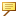About Operators Used in Relations
The following operators can be used in relations, both in equations and in conditional statements.
Arithmetic Operators
 + Addition – Subtraction / Division * Multiplication ^ Exponentiation () Parentheses for grouping for example, d0 = (d1–d2)*d3
Assignment Operator
 = Equal to
The equal (=) sign is an assignment operator that equates the two sides of an equation or relation. When the equal sign is used, the equation can have only a single parameter on the left side.
Comparison Operators
Comparison operators are used when a TRUE/FALSE value can be returned. For example, the following relation returns TRUE whenever d1 is greater than or equal to 3.5. It returns FALSE whenever d1 is less than 3.5:
d1 >= 3.5
The following comparison operators are supported:
 == Equal to > Greater than > = Greater than or equal to !=, <>,~= Not equal to < Less than <= Less than or equal to | Or & And ~, ! NotThe "equal to" assignment operator is different from the "equal to" comparison operator.
The operators |, &, !, and ~ extend the use of comparison relations by enabling several conditions to be set in a single statement. For example, the following relation returns TRUE whenever d1 is between 2 and 3, but not equal to 2.5:
d1 > 2 & d1 < 3 & d1 ~= 2.5